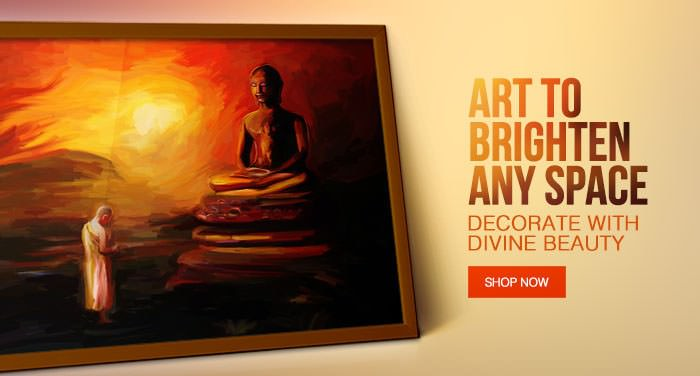Subscribe to RSS Feed

Jain ArtItems 21 to 40 of 143 total

Set Ascending Direction
per page

Grid  List

Page:

Starting at
Rs. 1,700.00

Starting at
Rs. 1,200.00

Starting at
Rs. 1,700.00

Starting at
Rs. 1,200.00

Starting at
Rs. 1,200.00

Starting at
Rs. 1,200.00

Starting at
Rs. 1,200.00

Starting at
Rs. 1,200.00

Starting at
Rs. 1,200.00

Starting at
Rs. 1,200.00

Starting at
Rs. 1,200.00

Starting at
Rs. 2,100.00

Starting at
Rs. 2,100.00

Starting at
Rs. 2,100.00

Starting at
Rs. 1,200.00

Starting at
Rs. 1,700.00

Starting at
Rs. 1,700.00

Starting at
Rs. 1,200.00

Starting at
Rs. 1,200.00
• What Is Unseen Is Eternal

Starting at
Rs. 1,700.00

Items 21 to 40 of 143 total

Set Ascending Direction
per page

Grid  List

Page: